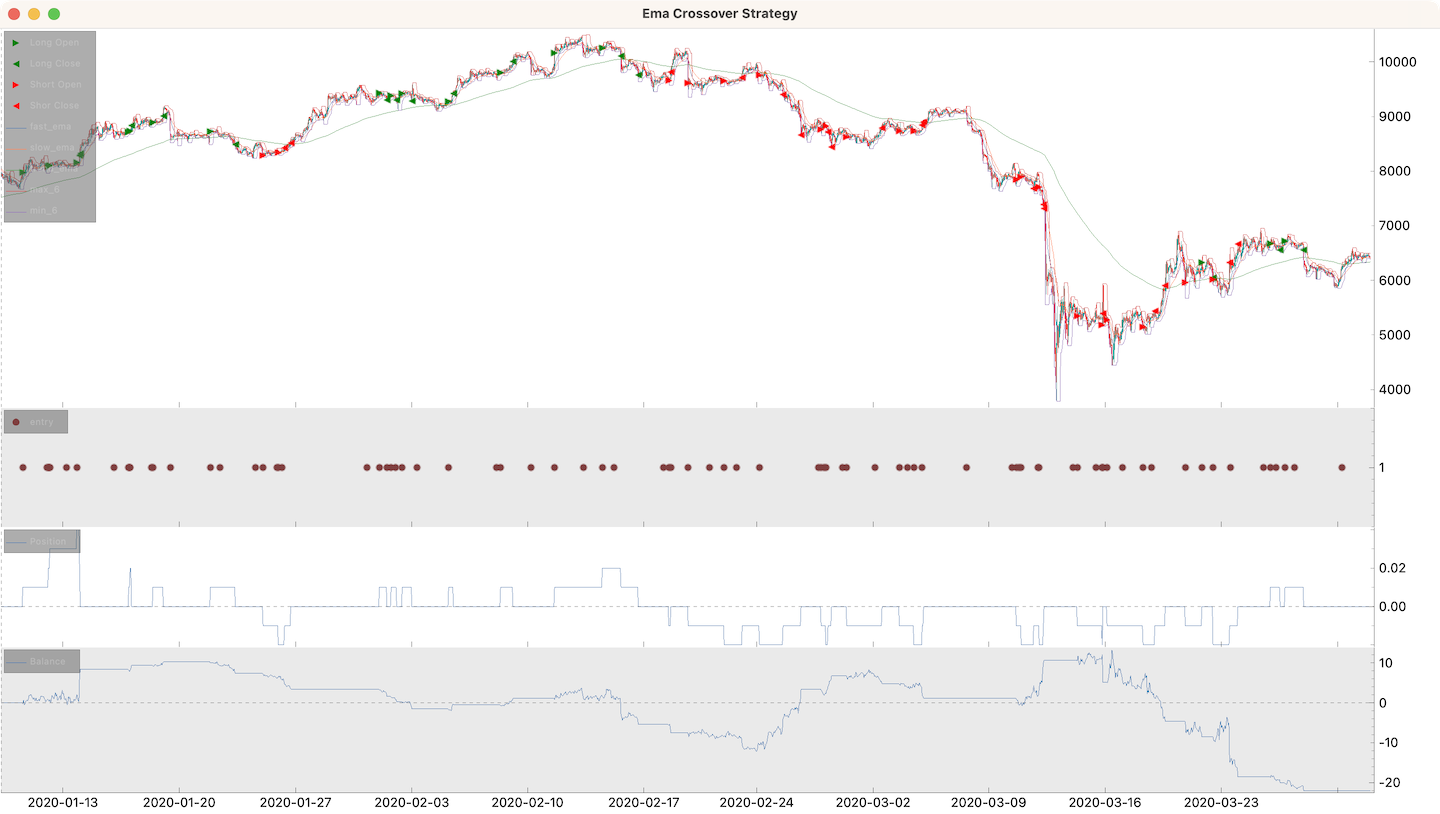# Introduction

Cipher is distinct from most other backtesting frameworks. It focuses on position adjustments in the scope of a session.

Translate to Cipher:

• `buy` -> `add to position`
• `sell` -> `reduce position`
• `trade` -> `session` (trading session)
• `close trade` -> `close session` (a side effect of adjusting position to 0)
• `order(market)` -> `transaction` (a side effect for position change)
• `order(limit, stop_loss)` -> `brackets`

## Features

• well-structured, simple to use, extensible
• multiple trading sessions at the same time
• complex exit strategies can be implemented (trailing take profit, etc.)
• multiple data sources support (multiple exchanges, symbols, timeframes, etc.)
• signal generation and signal handlers are splitted
• simple to run, just `python my_strategy.py`
• finplot and mplfinance plotters
• TA: pandas-ta is included, you can still use your libraries of choice

Cipher does no forward testing, paper, or live trading.

## Example

EMA crossover strategy example:

``````import numpy as np

from cipher import Cipher, Session, Strategy

class EmaCrossoverStrategy(Strategy):
def __init__(self, fast_ema_length=9, slow_ema_length=21, trend_ema_length=200):
self.fast_ema_length = fast_ema_length
self.slow_ema_length = slow_ema_length
self.trend_ema_length = trend_ema_length

def compose(self):
df = self.datas.df
df["fast_ema"] = df.ta.ema(length=self.fast_ema_length)
df["slow_ema"] = df.ta.ema(length=self.slow_ema_length)
df["trend_ema"] = df.ta.ema(length=self.trend_ema_length)

df["difference"] = df["fast_ema"] - df["slow_ema"]

# signal columns have to be boolean type
df["entry"] = np.sign(df["difference"].shift(1)) != np.sign(df["difference"])

df["max_6"] = df["high"].rolling(window=6).max()
df["min_6"] = df["low"].rolling(window=6).min()

return df

def on_entry(self, row: dict, session: Session):
if row["difference"] > 0 and row["close"] > row["trend_ema"]:
# start a new long session
session.position += "0.01"
session.stop_loss = row["min_6"]
session.take_profit = row["close"] + 1.5 * (row["close"] - row["min_6"])

elif row["difference"] < 0 and row["close"] < row["trend_ema"]:
# start a new short session
session.position -= "0.01"
session.stop_loss = row["max_6"]
session.take_profit = row["close"] - 1.5 * (row["max_6"] - row["close"])

# def on_<signal>(self, row: dict, session: Session) -> None:
#     """Custom signal handler, called for each open session.
#     # session.position = 1
#     # session.position = base(1)  # same as the one above
#     # session.position = '1'  # int, str, float are being converted to Decimal
#     # session.position = quote(100)  # sets position worth 100 quote asset
#     # session.position += 1  # adds to the position
#     # session.position -= Decimal('1.25')  # reduces position by 1.25
#     # session.position += percent(50)  # adds 50% more position
#     # session.position *= 1.5  # has the same effect as the one above
#     pass
#
# def on_take_profit(self, row: dict, session: Session) -> None:
#     """Called once take profit hit, default action - close position.
#     We can adjust the position and brackets here and let the session continue."""
#     session.position = 0
#
# def on_stop_loss(self, row: dict, session: Session) -> None:
#     """Called once stop loss hit, default action - close position.
#     We can adjust the position and brackets here and let the session continue."""
#     session.position = 0
#
# def on_stop(self, row: dict, session: Session) -> None:
#     """Called for each open session when the dataframe end reached.
#     We have an opportunity to close open sessions, otherwise - they will be ignored."""
#     session.position = 0

def main():
cipher = Cipher()
cipher.set_strategy(EmaCrossoverStrategy())
cipher.run(start_ts="2020-01-01", stop_ts="2020-04-01")
cipher.set_commission("0.00075")
print(cipher.sessions)
print(cipher.stats)
cipher.plot()

if __name__ == "__main__":
main()
``````## Disclaimer

This software is for educational purposes only. Do not risk money which you are afraid to lose. USE THE SOFTWARE AT YOUR OWN RISK. THE AUTHORS AND ALL AFFILIATES ASSUME NO RESPONSIBILITY FOR YOUR TRADING RESULTS.